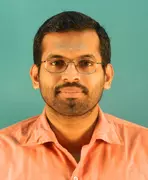X
On the complexity of l-reachability
K. S. Sunil
Published in IOS Press
2016
Volume: 145

Issue: 4
Pages: 471 - 483
Abstract
We initiate a complexity theoretic study of the language based graph reachability problem (L-REACH) : Fix a language L. Given a graph whose edges are labelled with alphabet symbols of the language L and two special vertices s and t, test if there is path P from s to t in the graph such that the concatenation of the symbols seen from s to t in the path P forms a string in the language L. We study variants of this problem with different graph classes and different language classes and obtain complexity theoretic characterizations for all of them. Our main results are the following: • Restricting the language using formal language theory we show that the complexity of L-REACH increases with the power of the formal language class. We show that there is a regular language for which the L-REACH is NL-complete even for undirected graphs. In the case of linear languages, the complexity of L-REACH does not go beyond the complexity of L itself. Further, there is a deterministic context-free language L for which L-DAGREACH is LogCFL-complete. • We use L-REACH as a lens to study structural complexity. In this direction we show that there is a language A in TC0 for which A-DAGREACH is NP-complete. Using this we show that P vs NP question is equivalent to P vs DAGREACH-1(P)1 question. This leads to the intriguing possibility that by proving DAGREACH-1(P) is contained in some subclass of P, we can prove an upward translation of separation of complexity classes. Note that we do not know a way to upward translate the separation of complexity classes.
Journal Fundamenta Informaticae IOS Press 01692968 Yes
Authors (1)
•Concepts (13)
•Computational complexity
•Computational linguistics
•Formal languages
•Translation (languages)
•ALPHABET SYMBOLS
•CFL REACHABILITY
•Complexity class
•DETERMINISTIC-CONTEXT-FREE-LANGUAGES
•FORMAL LANGUAGE THEORY
•Reachability
•Reachability problem
•Structural complexity
•Context free languages